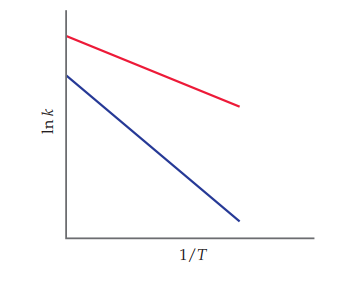×
Get Full Access to Chemistry: The Central Science - 14 Edition - Chapter 14 - Problem 14.10
Get Full Access to Chemistry: The Central Science - 14 Edition - Chapter 14 - Problem 14.10

×

# ?The accompanying graph shows plots of ln k versus 1/T for two different reactions. The plots have been extrapolated to the y-intercepts. Which reactioISBN: 9780134414232 1274

## Solution for problem 14.10 Chapter 14

Chemistry: The Central Science | 14th Edition

• Textbook Solutions
• 2901 Step-by-step solutions solved by professors and subject experts
• Get 24/7 help from StudySoup virtual teaching assistantsChemistry: The Central Science | 14th Edition

4 5 1 405 Reviews
24
4
Problem 14.10

The accompanying graph shows plots of ln k versus 1/T for two different reactions. The plots have been extrapolated to the y-intercepts. Which reaction (red or blue) has

(a) the larger value for $$E_{a}$$, and

(b) the larger value for the frequency factor, A? [Section 14.5]Text Transcription:

E_{a}

Step-by-Step Solution:

Step 1 of 5) Solubility is affected by temperature and by the presence of other solutes. The presence of an acid, for example, can have a major influence on the solubility of a substance. In Section 17.4, we considered the dissolving of ionic compounds in pure water. In this section, we examine three factors that affect the solubility of ionic compounds: (1) presence of common ions, (2) solution pH, and (3) presence of complexing agents. We will also examine the phenomenon of amphoterism, which is related to the effects of both pH and complexing agents.This reduction in solubility is another manifestation of the common-ion effect we looked at in Section 17.1. In general, the solubility of a slightly soluble salt is decreased by the presence of a second solute that furnishes a common ion, as Figure 17.17 shows for CaF2. The solubility of almost any ionic compound is affected if the solution is made sufficiently acidic or basic. The effects are noticeable, however, only when one (or both) ions in the compound is at least moderately acidic or basic. The metal hydroxides, such as Mg1OH22, are examples of compounds containing a strongly basic ion, the hydroxide ion. Let’s look at Mg1OH22, for which the solubility equilibrium.

Step 2 of 2

##### ISBN: 9780134414232

Since the solution to 14.10 from 14 chapter was answered, more than 214 students have viewed the full step-by-step answer. Chemistry: The Central Science was written by and is associated to the ISBN: 9780134414232. This full solution covers the following key subjects: . This expansive textbook survival guide covers 29 chapters, and 2820 solutions. The full step-by-step solution to problem: 14.10 from chapter: 14 was answered by , our top Chemistry solution expert on 10/03/18, 06:29PM. This textbook survival guide was created for the textbook: Chemistry: The Central Science, edition: 14. The answer to “?The accompanying graph shows plots of ln k versus 1/T for two different reactions. The plots have been extrapolated to the y-intercepts. Which reaction (red or blue) has (a) the larger value for $$E_{a}$$, and (b) the larger value for the frequency factor, A? [Section 14.5] Text Transcription:E_{a}” is broken down into a number of easy to follow steps, and 48 words.

## Discover and learn what students are asking

Calculus: Early Transcendental Functions : Second-Order Homogeneous Linear Equations
?Finding a General Solution In Exercises 5-30,find the general solution of the linear differential equation. y’’ - y' = 0

Unlock Textbook Solution